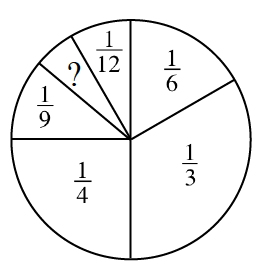Home > CC2 > Chapter 5 > Lesson 5.2.4 > Problem5-63

5-63.

Robert found an old game in a closet and wanted to play it. However, a portion of the spinner shown below could not be read. Find the missing portion of the spinner for Robert. Homework Help ✎Since the spinner must land on any of the portions, all of the portions must add up to $1$ because each of the portions is relative to its probability.

Try finding the difference between $1$ and the given probability portions. Since they have different denominators, you must find a common denominator for all of them and convert them to an equivalent fraction.

$\frac{2}{36}$ or $\frac{1}{18}$